Differences between revisions 12 and 13

 Deletions are marked like this. Additions are marked like this. Line 52: Line 52: == Any Extras == == Stopping down the telephoto lens ==

# Assignment 3 Camera Simulation

## Compound Lens Simulator

### Description of implementation approach and comments

After reading the lens data into a vector, I precomputed certain calculations for each lens (the z-index, the zMin and the zMax, etc.) so that they would not have to be computed each time whenever GenerateRay was called. I also computed the ratio by which the raster coordinates and the film coordinates are related (by dividing the given film diagonal (mm) with the diagonal calculated using the film's pixel resolution (pixels).

In GenerateRay, my algorithm works by converting the sample x and y coordinates into film coordinates and then choosing a point on the aperture of the backmost lens as the destination for that ray. I then pass the ray into the lens system, where for each lens, I calculate the refraction. To do this, I have to find each ray-lens intersection, which is done by modeling each lens as a partial sphere. Hence I perform ray-sphere intersection and use a z-min and z-max value in order to ensure that the intersection that I find will actually intersect the aperture of that lens. The ray is then refracted using a form of Snell's law (the vector form that can be found on Wikipedia). For the aperture stop, the effective aperture diameter is the parameter taken from the PBRT file. Here, I just perform a ray-plane intersection to make sure that the ray does not get stopped by the aperture, and I simulate the materials on either side of the aperture to be air (with a refractive index of 1). Any rays that are stopped anywhere along the lens system are given a weight of 0. All other rays have their weights calculated using the formula found in the Kolb paper. The area used is the area of the aperture of the backmost lens, the distance is the length between that aperture and the film plane, and the cosine is found by taking a dot product of the original angle of the ray with the z-axis.

### Final Images Rendered with 512 samples per pixel

 My Implementation Reference Telephoto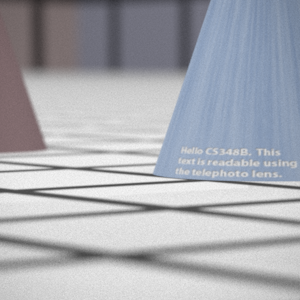Double Gausss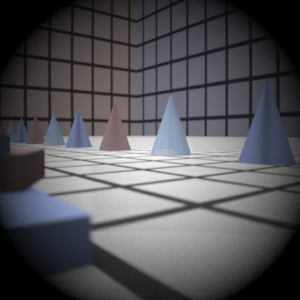Wide Angle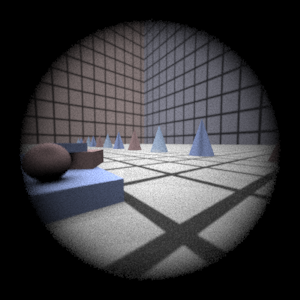Fisheye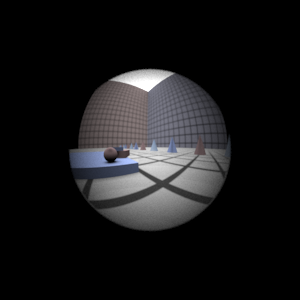### Final Images Rendered with 4 samples per pixel

 My Implementation Reference Telephoto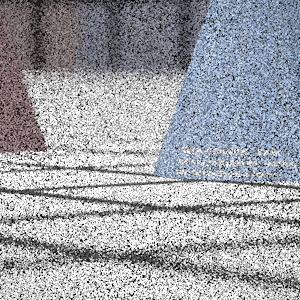Double Gausss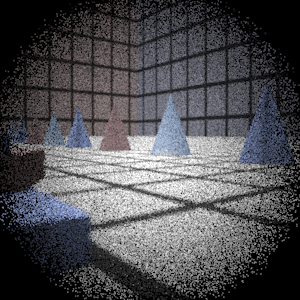Wide Angle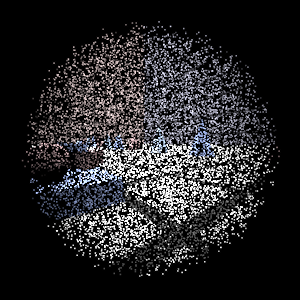Fisheye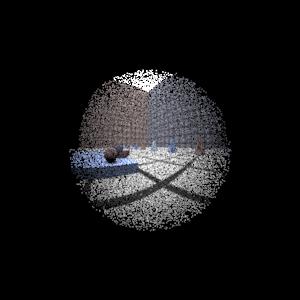## Experiment with Exposure

 Image with aperture full open Image with half radius aperture### Observation and Explanation

We expect the second image to be significantly darker than the first, because the smaller aperture lets in much less light. The image should be about 1/4 as bright, since the area of the second aperture is 1/4 the area of the first aperture. We've decreased the photo exposure by 2 stops by halving the aperture size.

## Autofocus Simulation

### Description of implementation approach and comments

In order to implement autofocus, my first step was to calculate the focal length of the given lens system. The focal length (f) is defined to be the distance between the lens system and the focal point (F2), where parallel rays coming from the world (representing rays coming from infinity) into the lens would converge. I calculate this by simulating parallel rays coming in and traversing the lens system backwards, and then calculating the intersection between these rays (after they've been refracted) and the z-axis. I do this by trying a small number of sample points in the world; if I do not successfully calculate the focal length, then I just start my search at zero. The advantage to calculating the focal length is that we know the filmdistance must be greater than or equal to the focal length, because the film plane must lie behind the focal point. The focal point and focal length is shown in this diagram:While using the focal length is of course good since it reduces the range to search, it's especially advantageous because the values of the SML function at very close distances (those closer than the focal length) are quite noisy and are frequently higher than the peak that ultimately indicates the well-focused image.

To find the optimal filmdistance, I used the Sum-Modified Laplacian as the focus measure function, as given in the suggested paper. My algorithm for evaluating the focus of a particular filmdistance would collect the RGB values on a CameraSensor, and then it would iterate through the pixel area, calculating the SML with a step size of 1 and using (R+G+B)/3 as the intensity formula. The SML is then the sum of all the ML values of the pixels in the area. I use the SML directly as a measure of the focus, because the SML acts as an edge detector, so high values are correlated with more precise edges (hence an in-focus image). The task then is to find the peak SML value and use the filmdistance that it corresponds to. Starting from the focal length as the minimum filmdistance value being tested, I try increasing filmdistances, recording the highest peak I've seen so far. I ended up using a step size of 5 mm in this step; although I tried out larger values (9, 10 and higher), I opted not to use them because the peaks are very narrow and are easily missed when using those larger ranges. I terminate the first pass after either searching to the upper limit of my range (250mm) or after I've encountered 10 monotonically decreasing values, hence indicating that I've already passed the peak. I then perform two more passes. On the second pass, I search in the vicinity of the highest peak found, this time traversing with a step size of 1mm and terminating after I see five monotonically decreasing values. On the third pass, I try to find the optimal focal distance at a really fine grain, I search with a step size of .1mm and search again in the vicinity of the highest peak found. I use this as my final value for filmdistance.

### Final Images Rendered with 512 samples per pixel

 Adjusted film distance My Implementation Reference Double Gausss 1 61.98 mm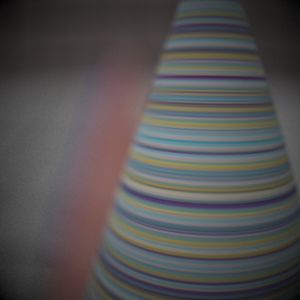Double Gausss 2 39.8 mmTelephoto 117.1 mm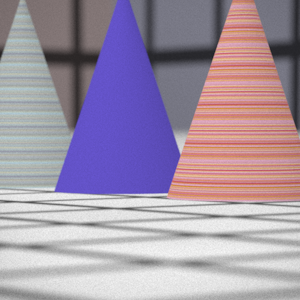## Stopping down the telephoto lensThe left image is the image that resulted from stopping down the telephoto lens by four stops. As expected, the image is significantly darker. However, in Photoshop, if you increase the exposure of the darker image by four (as seen in the rightmost image) and compare this image with the normal telephoto image, the result is clearly not the same.

The reason for this is that we are shooting the same number of rays per pixel when rendering each image, but when the aperture is smaller, many more rays are discarded. Hence the image rendered with the smaller aperture ends up being much noisier, since it has lost a lot of valuable data in discarded rays.

JulieTung/Assignment3 (last edited 2006-05-09 05:56:54 by JulieTung)